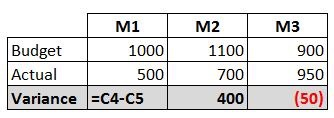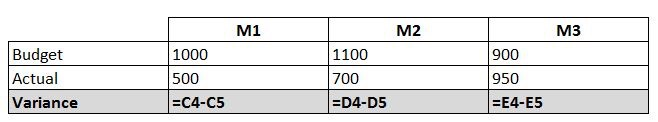This tip enables you to make an exact copy of a range of formulas without changing cell references or links to other workbooks.

When you copy a formula, Excel rather cleverly shifts the cells that the formula references.This is normally what you want it to do.So for example, if I have a table with a formula to calculate the monthly expenditure variance, I want to be able to copy the formula across so that it references the next column of data along.

A standard copy and paste will do this perfectly.But sometimes, I want to paste an exact copy of a range of formulas.

I spent a lot of time yesterday on somebody else’s model, only to be issued with a revision late in the day.

I wanted to replicate my changes without too much effort which meant pasting across a series of formulas that referenced a pivot table in the originating spreadsheet. Copying the formulas across in the normal copy and paste manner, created an external link to the original pivot table while I wanted it to refer to the pivot table in the revised workbook.

The ability to paste exact formulas was required.

Thankfully you can do this easily with the use of notepad to paste as text and then fooling excel to read this as a formula again.

1. Start in the worksheet with the formulas you want to copy1. Switch to Formula View Mode by using the shortcut Ctrl ` (note the use of the backward apostrophe which is under the escape key on my keyboard).
2. Copy the formulas